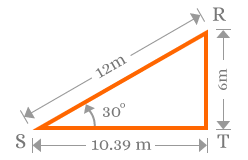# Sine values for angles

Ratio of length of the opposite side to length of the hypotenuse of a right angled triangle at an angle is defined, the value of sine for the angle.

The length of the opposite side and the length of the hypotenuse of a right angled triangle for an angle can be anything but the ratio of length of the opposite side to length of the hypotenuse is constant for the angle.

Consider a right angled triangle $\Delta RST$. Measure lengths of all three sides and also measure angle of the triangle. It is measured that lengths of the opposite side, adjacent side and hypotenuse are $6$$,$ $10.39$ and $12$ meters respectively. It is also measured that the angle of the right angled triangle is ${30}^{°}$.The value of the sine function at angle ${30}^{°}$ is calculated by calculating the ratio of length of the opposite side to length of the hypotenuse. At ${30}^{°}$, the length of the opposite side and length of the hypotenuse are $6$ meters and $12$ meters.

$sin{30}^{°}=\frac{6}{12}=0.5$

At the angle ${30}^{°}$, the value of the sine is equal to $0.5$.

Consider another right angled triangle $\Delta EFG$. Repeat the same procedure. It is measured that the length of the opposite side, adjacent side and hypotenuse are $10$$,$ $17.32$ and $20$ meters respectively. The angle of the right angled triangle is also 30. Now, calculate the value of sine at ${30}^{°}$.$sin{30}^{°}=\frac{10}{20}=0.5$

The lengths of opposite side and hypotenuses of both right angled triangles are different but angles of both right angled triangles are same. Similarly, the ratio of length of the opposite side to length of the hypotenuse is also same in both cases.

Latest Math Topics
Latest Math Problems
Email subscription
Math Doubts is a best place to learn mathematics and from basics to advanced scientific level for students, teachers and researchers. Know more